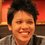# Fun ways to find the bounds of $\pi^2$ using Riemann Zeta Functions!

We all know that $\displaystyle \sum_{n=1}^\infty \frac1{n(n+1)} =\sum_{n=1}^\infty \left(\frac1n -\frac1{n+1}\right) = 1$ by partial fractions followed by telescoping sum. But if we raise the expression $\frac1{n(n+1)}$ to the power of any positive number greater than 1, something interesting happens!

By partial fractions, $\left(\frac1{n(n+1)}\right)^3 = \left(\frac1{n^3} - \frac1{(n+1)^3}\right) - \left(\frac1{n^2} + \frac1{(n+1)^2} \right) - 6 \left(\frac1n - \frac1{n+1} \right)$.

With the knowledge of the Basel function: $\displaystyle \sum_{n=1}^\infty \frac1{n^2} = \frac{\pi^2}6$.

\begin{aligned} &&\displaystyle \sum_{n=1}^\infty \left(\frac1{n(n+1)}\right)^3 \\ &=& \displaystyle \sum_{n=1}^\infty \left[ \left(\frac1{n^3} - \frac1{(n+1)^3}\right) - 3\left(\frac1{n^2} + \frac1{(n+1)^2} \right) + 6 \left(\frac1n - \frac1{n+1} \right) \right ] \\ &=& \displaystyle (1) - 3 \left( 2\left( \frac{\pi^2}{6}\right) - 1\right) + 6(1) \\ &=& 10 - \pi^2 \\ \end{aligned}

Because $\displaystyle \sum_{n=1}^\infty \left(\frac1{n(n+1)}\right)^3$ is the sum of all positive terms, then it must be strictly positive, then $10 - \pi^2 > 0$, equivalently $\pi^2 < 10$. Thus we just found an upper bound of $\pi^2$. Wonderful isn't it?

You could keep increasing the value of $m$ for the expression $\left(\frac1{n(n+1)}\right)^m$ for positive number $m$ to increase the accuracy of the bound!

Try it yourself!

Question 1: Prove the partial fraction $\left ( \frac1{n(n+1)} \right)^2 \equiv \left ( \frac 1{n^2} + \frac1{(n+1)^2} \right) - 2 \left (\frac1n -\frac1{n+1}\right)$. And determine the value of $\displaystyle \sum_{n=1}^\infty \left ( \frac1{n(n+1)} \right)^2$. Thus show that $\pi > 3$.

Question 2: Given that $\displaystyle \sum_{n=1}^\infty \frac1{n^4} = \frac{\pi^4}{90}$ and find the partial fraction of $\left(\frac1{n(n+1)}\right)^5$, prove that $\pi^2 < \frac32 \left(\sqrt{1729}-35\right)$. Consider the example given and the answer you've found, can you determine a systematic way to increase the upper bound of $\pi^2$?

Question 3: Suppose we restrict $m$ (as mentioned above) to an odd number, why does the accuracy of the upper bound increases when the number $m$ increases?

Question 4: Consider $\displaystyle \sum_{n=1}^\infty \left( \frac1{n(n+1)(n+2)}\right)^2$, prove that $\pi> \frac{ \sqrt{39}}2$. Similarly, consider $\displaystyle \sum_{n=1}^\infty \left( \frac1{n(n+1)(n+2)(n+3)}\right)^2$, prove that $9.85 < \pi^2 < 10$.

Question 5 Using the answers you got from Question 2, find the partial fractions for $\left(\frac1{n(n+1)}\right)^4$, and thus prove the inequality $\large 15(4\sqrt2 -5) < \pi^2 < \frac32 \left(\sqrt{1729}-35\right).$

Question 6 (warning: tedious): Like in Question 4, consider $\displaystyle \sum_{n=1}^\infty \left( \frac1{n(n+1)(n+2)(n+3)(n+4)}\right)^2$, prove that $\pi^2 > \frac{1105}{112}$.

Question 7: From Question 4 and 6, can you spot a pattern to find a lower bound of $\pi^2$? Prove that using this technique you've found, you can always find a rational number $A$ such that $\pi^2 > A$.

Question 8 (warning: very tedious): If we are further given that $\displaystyle \zeta(6) = \sum_{n=1}^\infty \frac1{n^6} = \frac{\pi^6}{945}$, and find the partial fraction of $\left(\frac1{n(n+1)}\right)^7$ and by Cardano's method, prove that $\large \pi^2 < -42 + 3 \left ( \sqrt{7486 + \sqrt{62791465}} - \frac{189}{\sqrt{7486 + \sqrt{62791465}}} \right )$.

Question 9 (warning: extremely tedious): From Question 8, if we are again further given that $\zeta(8) = \frac{\pi^8}{9450}$, using the approaches we had before, prove that the equation below has a positive real root $B$ such that $B - \pi^2$ is a significantly small positive number.

$\large 3x^4 + 550x^3 + 45045x^2 + 3378375x - 38288250 = 0$Note by Pi Han Goh
5 years, 5 months ago

This discussion board is a place to discuss our Daily Challenges and the math and science related to those challenges. Explanations are more than just a solution — they should explain the steps and thinking strategies that you used to obtain the solution. Comments should further the discussion of math and science.

When posting on Brilliant:

• Use the emojis to react to an explanation, whether you're congratulating a job well done , or just really confused .
• Ask specific questions about the challenge or the steps in somebody's explanation. Well-posed questions can add a lot to the discussion, but posting "I don't understand!" doesn't help anyone.
• Try to contribute something new to the discussion, whether it is an extension, generalization or other idea related to the challenge.

MarkdownAppears as
*italics* or _italics_ italics
**bold** or __bold__ bold
- bulleted- list
• bulleted
• list
1. numbered2. list
1. numbered
2. list
Note: you must add a full line of space before and after lists for them to show up correctly
paragraph 1paragraph 2

paragraph 1

paragraph 2

[example link](https://brilliant.org)example link
> This is a quote
This is a quote
    # I indented these lines
# 4 spaces, and now they show
# up as a code block.

print "hello world"
# I indented these lines
# 4 spaces, and now they show
# up as a code block.

print "hello world"
MathAppears as
Remember to wrap math in $$ ... $$ or $ ... $ to ensure proper formatting.
2 \times 3 $2 \times 3$
2^{34} $2^{34}$
a_{i-1} $a_{i-1}$
\frac{2}{3} $\frac{2}{3}$
\sqrt{2} $\sqrt{2}$
\sum_{i=1}^3 $\sum_{i=1}^3$
\sin \theta $\sin \theta$
\boxed{123} $\boxed{123}$

Sort by:

If we continue to use this trick, will it eventually converge to pi ( or pi^2,pi^4 etc), or will it always be really close, but not pi itself?I doubt it, because every time we have an algebraic number as an approximation and pi is transcendental.

- 5 years, 5 months ago

Woah! I'll check this out soon :)

- 5 years, 5 months ago

I love it :) .........Sir finding an estimate for pi requires the zeta at even integers ..... can we reverse this method to instead approximate for zeta at odd integers?

- 5 years, 5 months ago

Cool! Please keep on sharing such awesome things like this with us .

- 5 years, 5 months ago

Just asking, is the polynomial in question $8$ wrong? I'm not sure about it but I graphed it out and there was no root near $\pi^{2}$. It may be possible that Desmos screwed up because the numbers are too big.

- 5 years, 5 months ago

- 5 years, 5 months ago

It's correct now... I think I made a mistake typing it last night.

- 5 years, 5 months ago